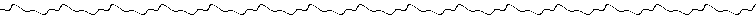LESSON4:

If.. Then.. Else statements allow the program to branch off in many different directions. ASM does not support these staments directly, but using CP along with JR/JPyou can acheive the effect.These are the instructions/ideas that will be covered in this lesson:

CP

IF... THEN...ELSECP
CP simply sets the FLAG register (F) based on the subraction (A-s)

• CP (where S = A,F,B,C,D,E,H,L,#,(hl),(iy+#),or (ix+#))

back to list of instructionsIF... THEN... ELSE
To acheivean IF... THEN... ELSE statement(s) you must use a CP call followed by aJR, JP, or any other instruction that has conditionals (ret, call, ...) Here are some examples:

ld b,5       ;b=5
ld a,5       ;a=5

cp b         ;set flags based on (a-b)
jr z,AequalsB ;if a=b -> a-b=0,therefore
:
.
AequalsB: ;
:
.

You can also string them together like this:

ld b,6  ;.
ld c,5  ;.
ld a,5  ;Set the init values

cp b    ;IF a=b
jr z,AB ;THEN goto AB
cp c    ;IF a=c
jr z,AC ;THEN goto AC
jr NONE ;ELSE goto NONE (if it didn'tjump one of the other
; times then it must be an ELSE, NOTE:no conditia
; listed.  Just a straight 'jr NONE')
:
.
AB:
:
.
AC:
:
.
NONE:

You've seen how to test for equality.  now for GREATER THAN, and LESS THAN:

• Compare A and B for =, < and >
LD B, 7
LD A, 5

CP B ; Flags = status(A-B)
JP Z, A_Equal_To_B ; IF(a-b)=0 THEN a=b
JP NC, A_Greater_Than_B ; IF(a-b)>0 THEN a>b
JP C, A_Lower_Than_B ; IF(a-b)<0 THEN a<b

A_Greater_Than_B:
(...)
JP end_compare

A_Lower_Than_B:
(...)
JP end_compare

A_Equal_To_B:
(...)

end_compare:

RET
END

:
:
.

back to list of instructionsLESSON3     INDEX     LESSON5

This is the end of the lesson, I do not guarantee any correctness of any statements made above.